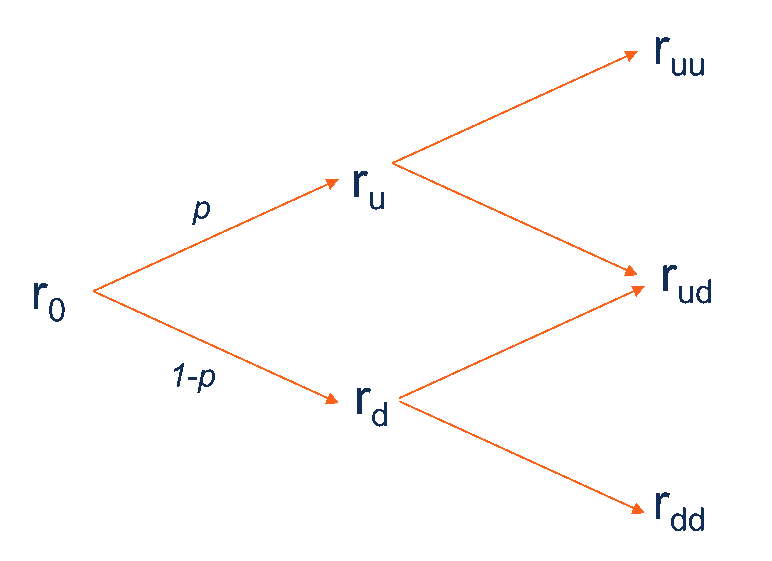# Binomial Interest Rate Tree

A graphical representation of possible interest rate values at different periods of time

## What is the Binomial Interest Rate Tree?

The binomial interest rate tree is a graphical representation of possible interest rate values at different periods of time under the assumption that at each time period, the interest rate may either increase or decrease with a certain probability. Essentially, the binomial interest rate tree concerns with the evolution of short-term interest rates.### Uses of the Binomial Interest Rate Tree

Binomial interest rate trees are primarily used to price bonds (including plain-vanilla bonds, callable bonds, and puttable bonds) and various derivatives whose payoffs are linked to the bonds. The concept of the binomial interest tree also comes with applications in other pricing models such as the Black-Derman-Toy model.

Generally, the binomial interest rate tree is a simple and easily understandable approach to project future interest rates that can be used to calculate the price of bonds or derivatives. However, the main idea of this model that the interest rate may either go up or down may not always properly work in the real world.

### How to Create the Binomial Interest Rate Tree?

The binomial interest rate tree can be created by following the next steps:

1. Observe the current interest rate of the interested security (bond or derivative).
2. Determine the probability of the interest rate either go up or down. In most cases, the risk-neutral probability (i.e., the probability of future outcomes adjusted for risk) is used to calculate the future interest rate. Note that if the probability of the interest rate increase equals p, the probability of the interest rate decrease equals (1-p). In addition, the risk-neutral probability can be used for calculating the future rates in all time periods.
3. Calculate the forward (future) rates using the determined probability.
4. Create the binomial tree using the obtained interest rates. The tree must look like the image above (the binomial interest rate tree for two periods).

Note that similar to other binomial trees, in the binomial interest rate tree, the current price of a bond or derivative must be calculated backward. In other words, firstly, we must calculate the prices of security in the latest periods and then calculate the prices in the previous periods.

### More Resources

CFI offers the Financial Modeling & Valuation Analyst (FMVA)™ certification program for those looking to take their careers to the next level. To keep learning and advancing your career, the following resources will be helpful:

• Coupon Rate
• Federal Reserve
• Floating Interest Rate
• Interest Rate Swap

### Financial Analyst Training

Get world-class financial training with CFI’s online certified financial analyst training program!

Gain the confidence you need to move up the ladder in a high powered corporate finance career path.

Learn financial modeling and valuation in Excel the easy way, with step-by-step training.